Скачать презентацию Appendix to Chapter 4 Demand Theory A Mathematical

• Количество слайдов: 15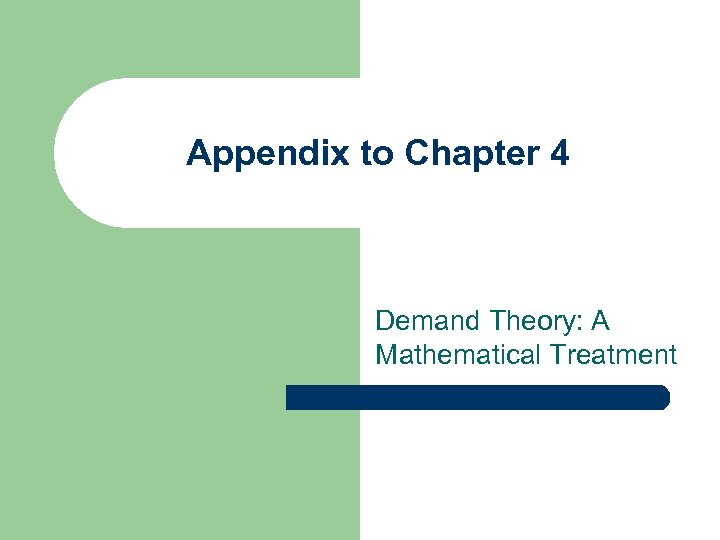Appendix to Chapter 4 Demand Theory: A Mathematical Treatment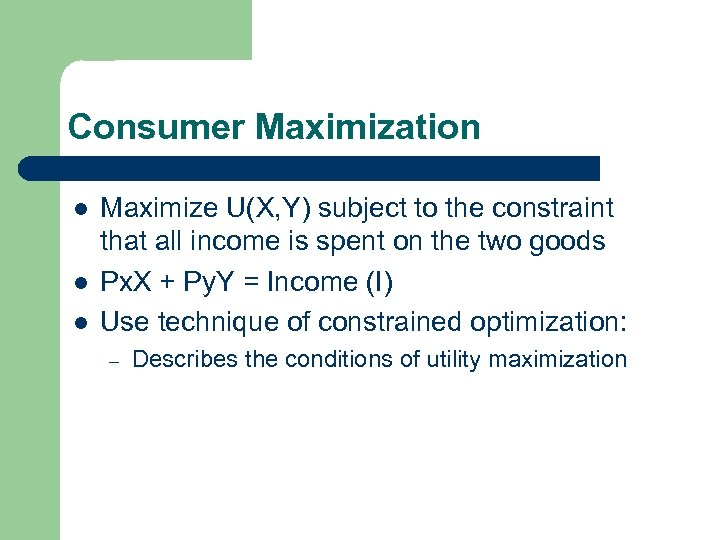Consumer Maximization l l l Maximize U(X, Y) subject to the constraint that all income is spent on the two goods Px. X + Py. Y = Income (I) Use technique of constrained optimization: – Describes the conditions of utility maximization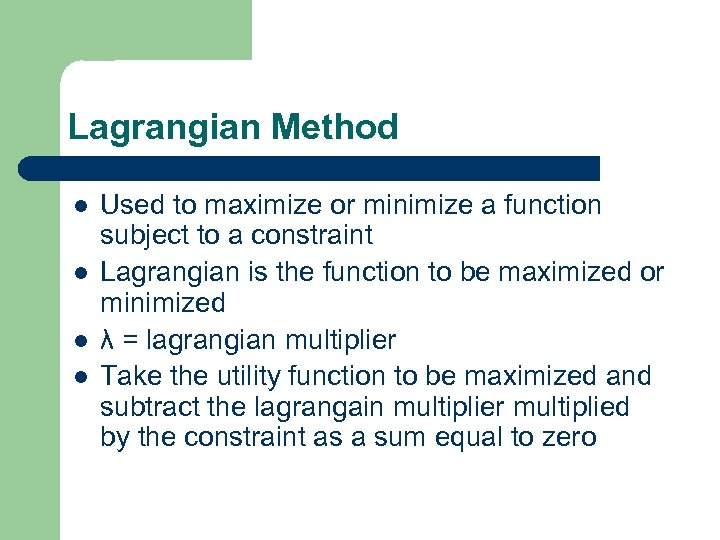Lagrangian Method l l Used to maximize or minimize a function subject to a constraint Lagrangian is the function to be maximized or minimized λ = lagrangian multiplier Take the utility function to be maximized and subtract the lagrangain multiplier multiplied by the constraint as a sum equal to zero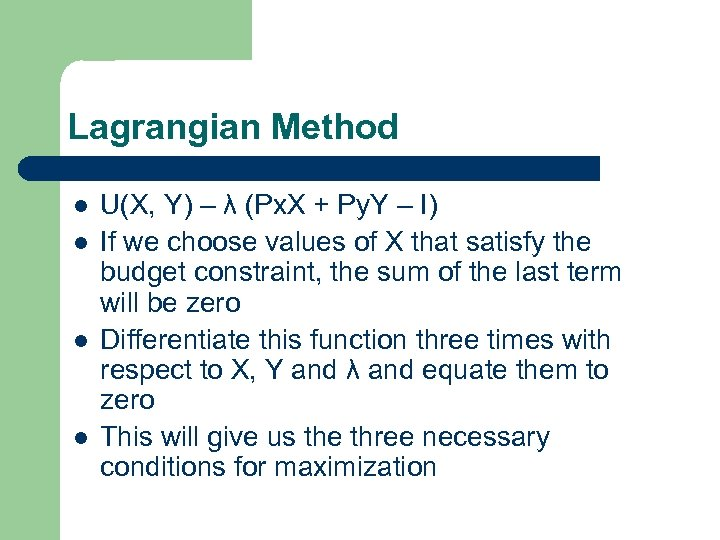Lagrangian Method l l U(X, Y) – λ (Px. X + Py. Y – I) If we choose values of X that satisfy the budget constraint, the sum of the last term will be zero Differentiate this function three times with respect to X, Y and λ and equate them to zero This will give us the three necessary conditions for maximization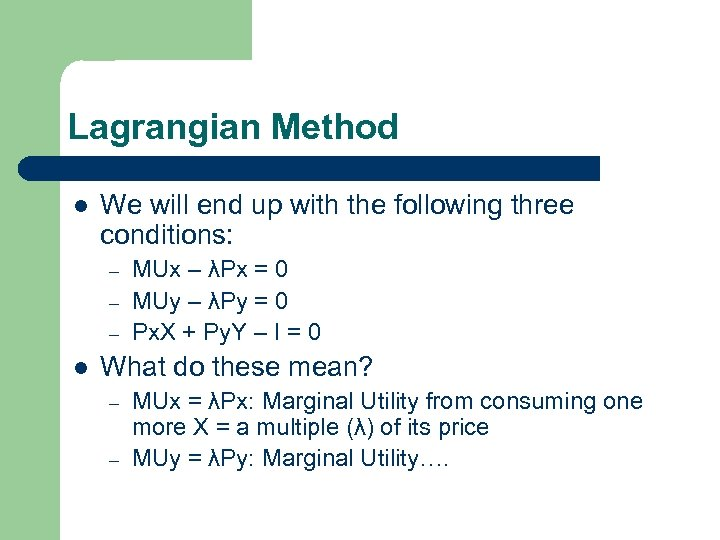Lagrangian Method l We will end up with the following three conditions: – – – l MUx – λPx = 0 MUy – λPy = 0 Px. X + Py. Y – I = 0 What do these mean? – – MUx = λPx: Marginal Utility from consuming one more X = a multiple (λ) of its price MUy = λPy: Marginal Utility….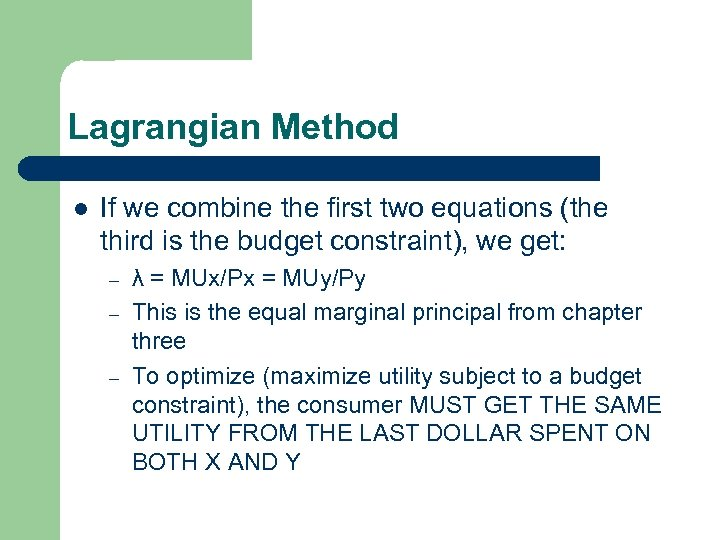Lagrangian Method l If we combine the first two equations (the third is the budget constraint), we get: – – – λ = MUx/Px = MUy/Py This is the equal marginal principal from chapter three To optimize (maximize utility subject to a budget constraint), the consumer MUST GET THE SAME UTILITY FROM THE LAST DOLLAR SPENT ON BOTH X AND Y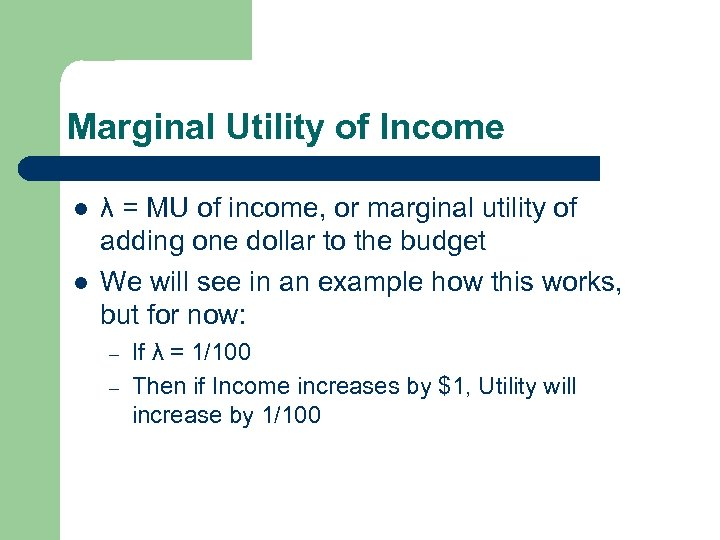Marginal Utility of Income l l λ = MU of income, or marginal utility of adding one dollar to the budget We will see in an example how this works, but for now: – – If λ = 1/100 Then if Income increases by \$1, Utility will increase by 1/100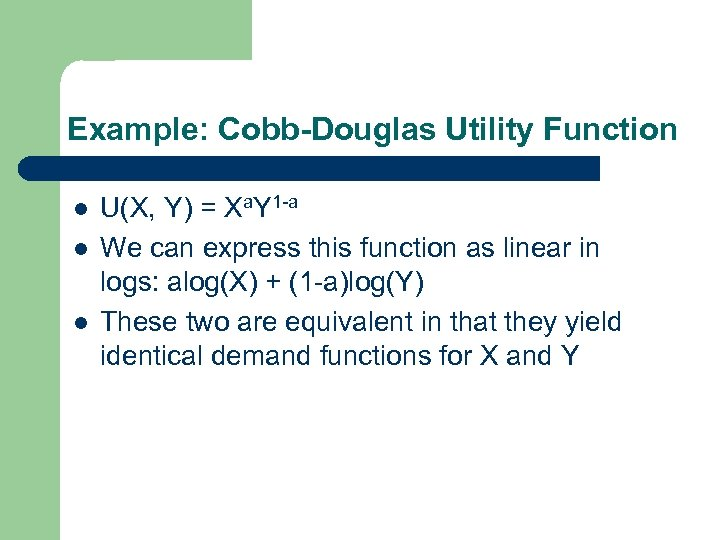Example: Cobb-Douglas Utility Function l l l U(X, Y) = Xa. Y 1 -a We can express this function as linear in logs: alog(X) + (1 -a)log(Y) These two are equivalent in that they yield identical demand functions for X and Y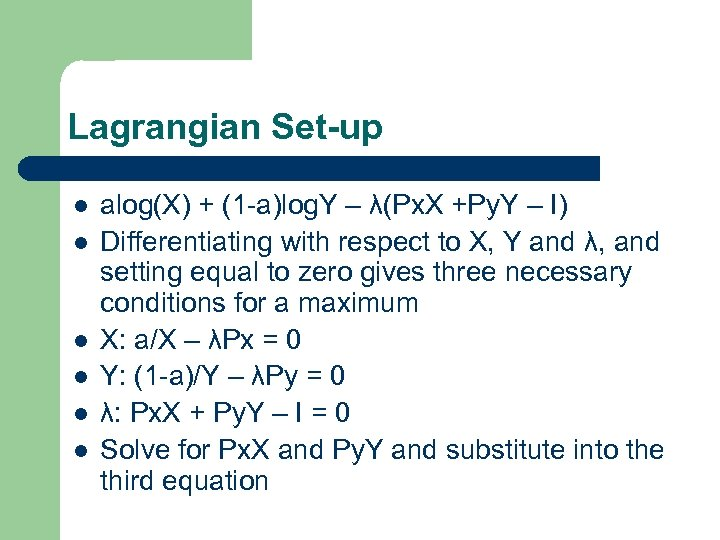Lagrangian Set-up l l l alog(X) + (1 -a)log. Y – λ(Px. X +Py. Y – I) Differentiating with respect to X, Y and λ, and setting equal to zero gives three necessary conditions for a maximum X: a/X – λPx = 0 Y: (1 -a)/Y – λPy = 0 λ: Px. X + Py. Y – I = 0 Solve for Px. X and Py. Y and substitute into the third equation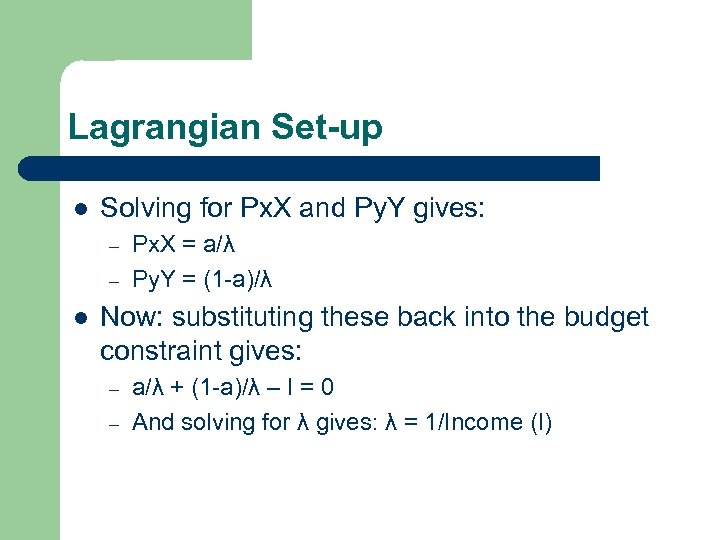Lagrangian Set-up l Solving for Px. X and Py. Y gives: – – l Px. X = a/λ Py. Y = (1 -a)/λ Now: substituting these back into the budget constraint gives: – – a/λ + (1 -a)/λ – I = 0 And solving for λ gives: λ = 1/Income (I)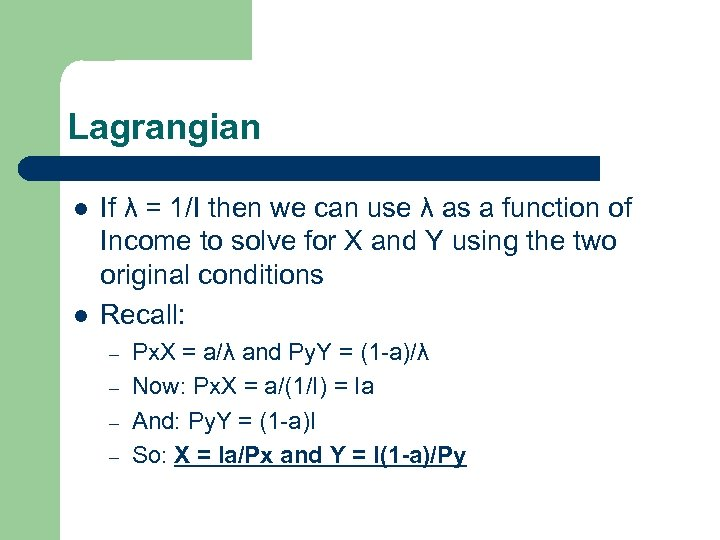Lagrangian l l If λ = 1/I then we can use λ as a function of Income to solve for X and Y using the two original conditions Recall: – – Px. X = a/λ and Py. Y = (1 -a)/λ Now: Px. X = a/(1/I) = Ia And: Py. Y = (1 -a)I So: X = Ia/Px and Y = I(1 -a)/Py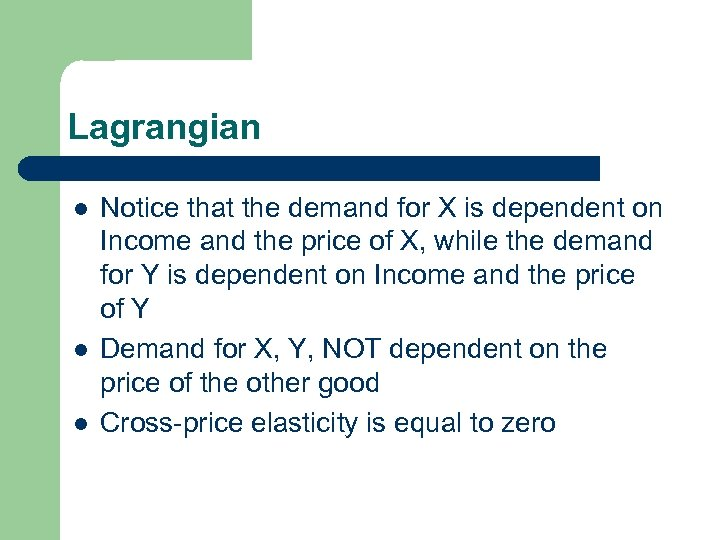Lagrangian l l l Notice that the demand for X is dependent on Income and the price of X, while the demand for Y is dependent on Income and the price of Y Demand for X, Y, NOT dependent on the price of the other good Cross-price elasticity is equal to zero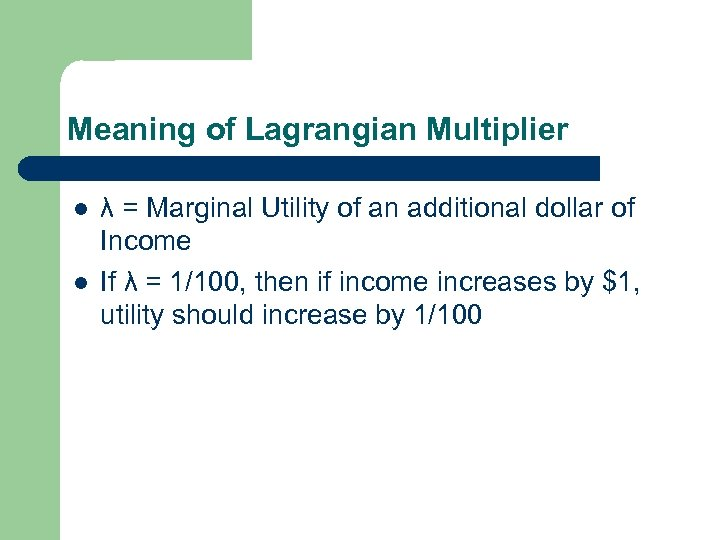Meaning of Lagrangian Multiplier l l λ = Marginal Utility of an additional dollar of Income If λ = 1/100, then if income increases by \$1, utility should increase by 1/100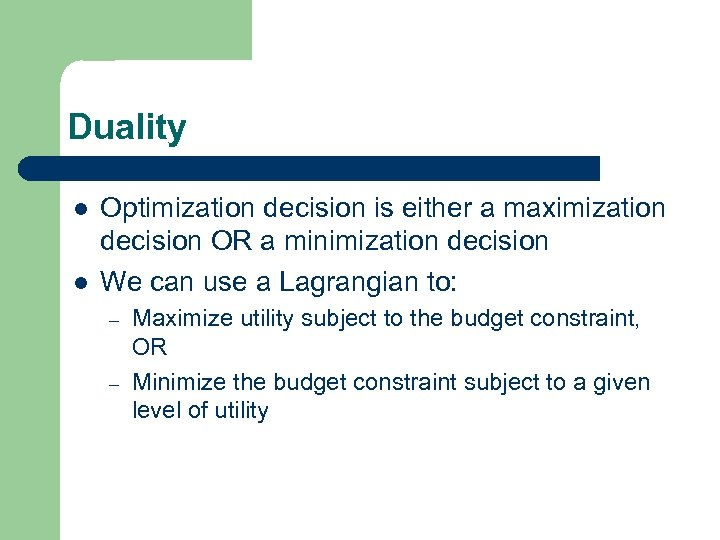Duality l l Optimization decision is either a maximization decision OR a minimization decision We can use a Lagrangian to: – – Maximize utility subject to the budget constraint, OR Minimize the budget constraint subject to a given level of utility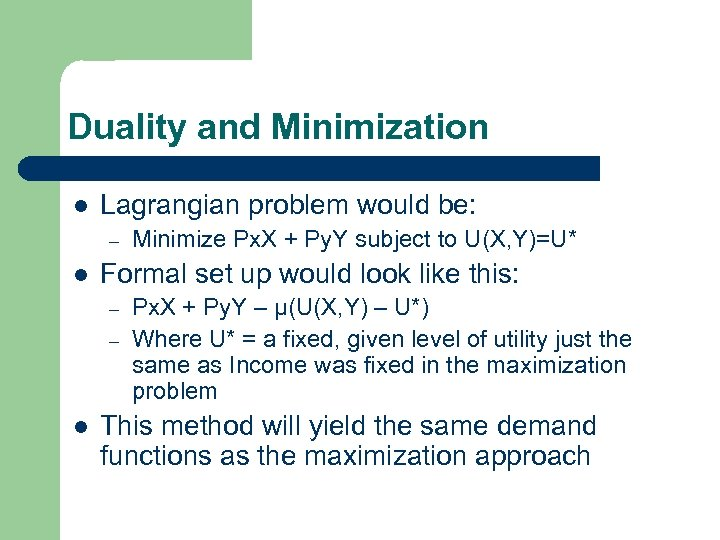Duality and Minimization l Lagrangian problem would be: – l Formal set up would look like this: – – l Minimize Px. X + Py. Y subject to U(X, Y)=U* Px. X + Py. Y – μ(U(X, Y) – U*) Where U* = a fixed, given level of utility just the same as Income was fixed in the maximization problem This method will yield the same demand functions as the maximization approach